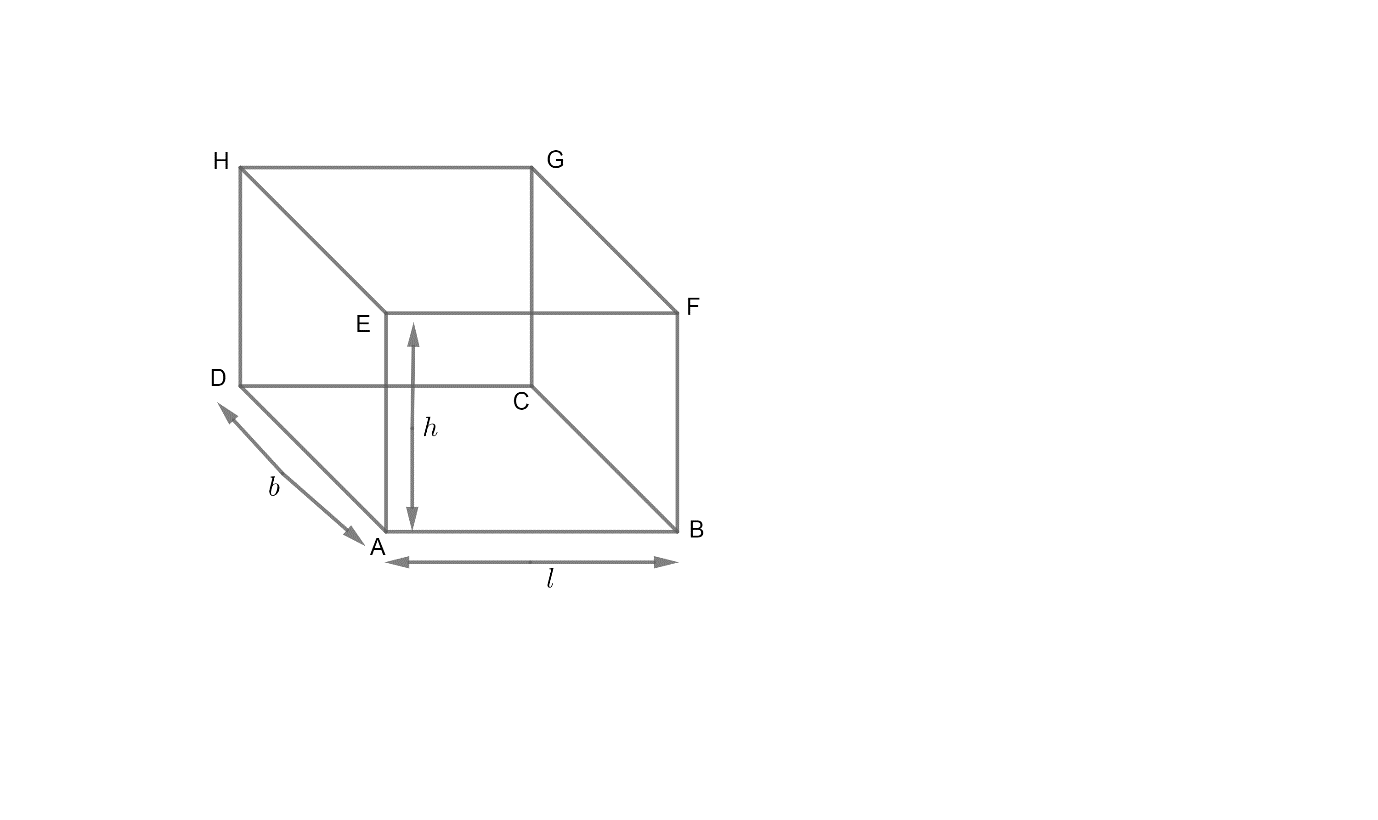QUESTION

# If $l,b,h$are the length, breadth and height of a room, then area of 4 walls will be,A. $2\left( l+b \right)h$B. $\left( l+b \right)h$C. $lbh$D. $2\left( lb+bh+hl \right)h$

Hint:If we look around and observe the walls of a room, we find that generally the walls are in the shape of a rectangle. The floor and the ceiling of the room are also of rectangular shape. We have a formula for finding the area of a rectangle.
Area of rectangle $=length\ \times \ breadth$
Apply this formula for the four walls separately and then add them to get the area of four walls.

Complete step-by-step solution -Suppose ABFE, BCGF, CDHG and DAEH are the four walls whose area we need to find.
Given,
$l,b\ and\ h$ are the length, breadth and height of a room.
So,
Length of AB $=l$
Length of AD $=b$
Length of AE $=h$
For the rectangle ABFE,
Length of AB $=l$
Length of AE $=h$
So, Area of rectangle ABFE $=AB\times AE$
$=\left( l\times h \right)$………….(1)
For the rectangle BCGF,
Length of BC = length of AD $=b$
Length of BF = length of AE $=h$
So, Area of rectangular wall BCGF
\begin{align} & =BC\times BF \\ & =\left( b\times h \right)...........\left( 2 \right) \\ \end{align}
For the rectangular wall CDHG,
Length of CD = length of AB $=l$
Length of CG = length of AE $=h$
So, Area of rectangular wall CDHG
\begin{align} & =CD\times CG \\ & =lh...........\left( 3 \right) \\ \end{align}
For the rectangular wall DAEH,
Length of AD $=b$
Length of AE $=h$
So, Area of rectangular wall DAEH
\begin{align} & =AD\times AE \\ & =b\times h.........\left( 4 \right) \\ \end{align}
Now, the total area of four walls = Area of wall ABFE + Area of wall BCGF + Area of wall CDHG +Area of wall DAEH
So, adding equations (1), (2), (3) and (4), we get,
Total Area of four walls $=\left( l\times h+b\times h+l\times h+b\times h \right)$
\begin{align} & =\left( lh+bh+lh+bh \right) \\ & =2lh+2bh \\ \end{align}
Area of four walls $=2lh+2bh$
Taking $2h$ common from both terms, we get,
Area of four walls $=2\left( l+b \right)h$
Hence, option (A) is correct.

Note: You can also do it quickly.
Look around in the room you are sitting to understand better. Observe that all four walls whose area we have to find have the $h$ (height of the room) as one side and other side will be length $\left( l \right)$ for two and breadth $\left( b \right)$ for the other two walls.
Also, observe the opposite walls being of the same size and shape and hence, have the same area too.
So,
Area of four walls $=2\left( lh \right)+2\left( bh \right)$
$=2\left( l+b \right)h$
(Taking $2h$ common from both terms).